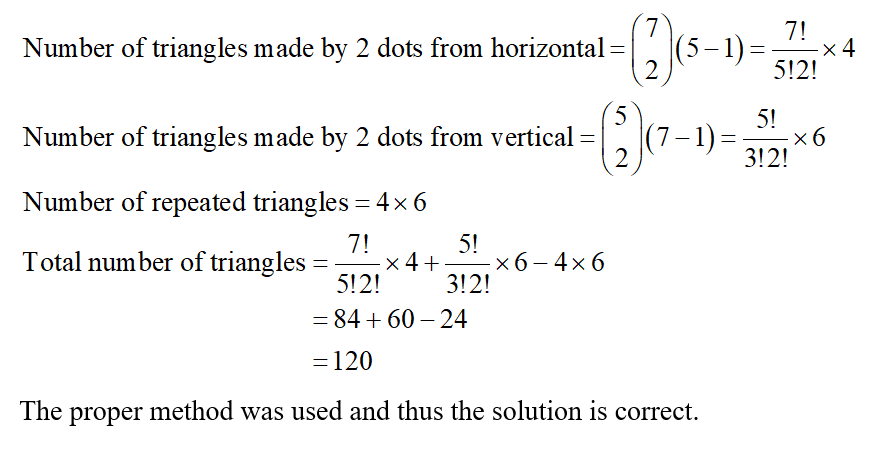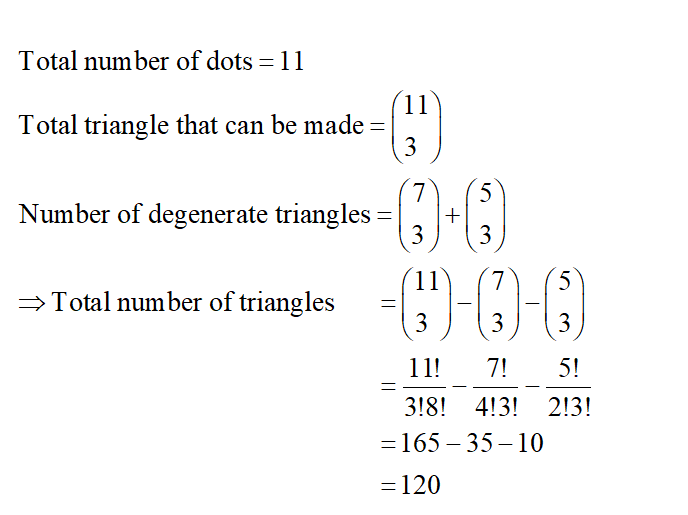# Consider all the triangles you can create using the points shown below as vertices. Note, we are not allowing degenerate triangles (ones with all three vertices on the same line) but we do allow non-right triangles. Find the number of triangles, and explain why your answer is correct.Find the number of triangles again, using a different method. Explain why your new method works.State a binomial identity that your two answers above establish (that is, give the binomial identity that your two answers a proof for). Then generalize this using mm's and nn's.

Question
84 views

Consider all the triangles you can create using the points shown below as vertices. Note, we are not allowing degenerate triangles (ones with all three vertices on the same line) but we do allow non-right triangles.

1. Find the number of triangles, and explain why your answer is correct.

2. Find the number of triangles again, using a different method. Explain why your new method works.

3. State a binomial identity that your two answers above establish (that is, give the binomial identity that your two answers a proof for). Then generalize this using mm's and nn's.

check_circle

Step 1

1. Obtain the number of triangles as follows.Step 2

2. Obtain the number of triangles as follows....

### Want to see the full answer?

See Solution

#### Want to see this answer and more?

Solutions are written by subject experts who are available 24/7. Questions are typically answered within 1 hour.*

See Solution
*Response times may vary by subject and question.
Tagged in

### Math